# Catch up on the latest AI articles# Accurate Modeling Of Human-like And Interestingness Of Generated Text: MAUVE

3 main points
✔️ Developed a method to automatically assess the human-like nature of raw codification
✔️ Modeled Type-I and Type-II errors using KL-divergence
✔️ Achieves the highest accuracy with a significantly higher correlation to manual assessment than existing methods

(Submitted on 2 Feb 2021 (v1), last revised 23 Nov 2021 (this version, v3))
NeurIPS 2021
Subjects: Computation and Language (cs.CL)code：The images used in this article are from the paper, the introductory slides, or were created based on them.

## first of all

There is a task called open-ended language generation. Some of the most famous ones are chat dialogue and story generation. Do you know how to evaluate those tasks automatically?

Machine translation and summarization have a clear correct answer, so we try to measure the distance from the correct answer by using BLEU or ROUGE. However, in In open-ended language generation, there is no clear correct answer. Therefore We use language models to indirectly estimate whether a sentence is human-like and interesting or not. The following metrics are introduced as baselines in this paper.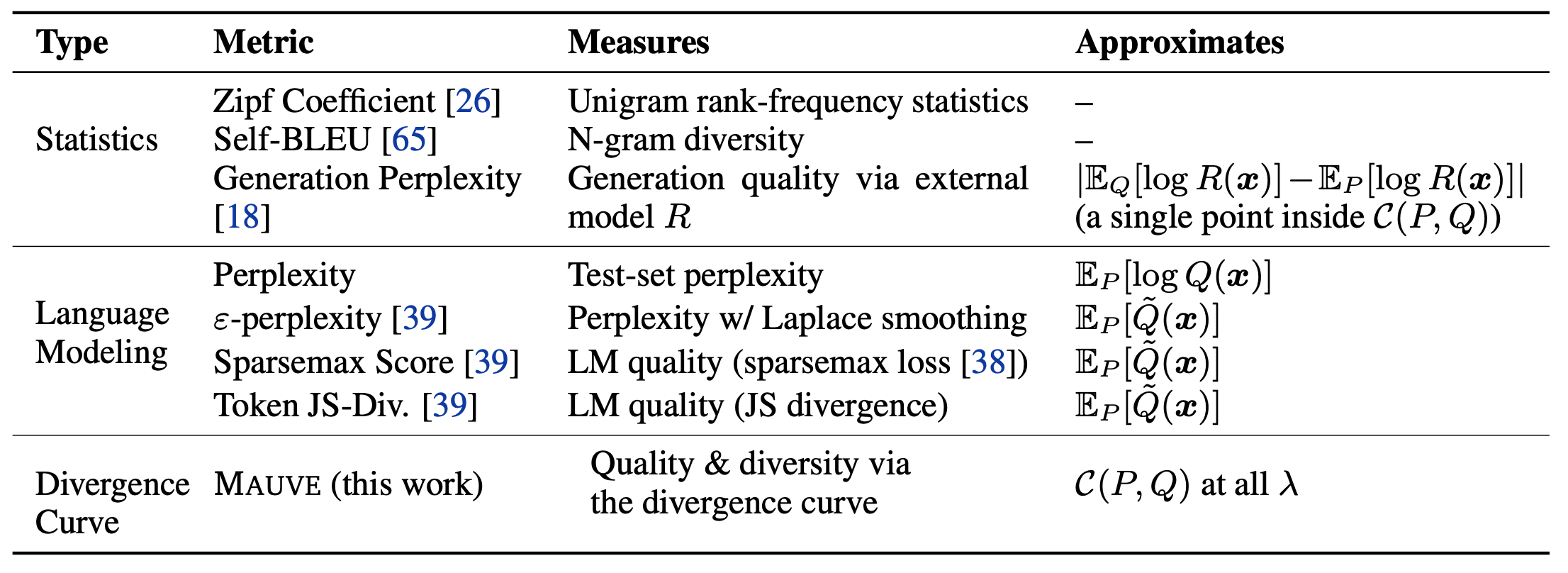The most famous one in the table is probably Perplexity. It evaluates the likelihood of the generated sentences. Self-BLEU evaluates the diversity by comparing the BLEU among the generated sentences. The proposed method MAUVE, which is shown at the bottom of the table, achieves a much higher correlation with human evaluation than those previous studies by using KL-divergence.

This article provides an overview of MAUVE, which was also selected as an Outstanding paper by NeurIPS.

## MAUVE

### Modeling of Type-I and Type-II Errors

The concepts of Type-I and Type-II errors are often used to classify errors in models. They are also referred to as False-positive and False-negative, respectively, and can be applied to language generation as

• Type-I error: generates sentences that are unlikely to be written by humans (repetition is a typical example)
• Type-II error: Unable to generate sentences that a human would write.

The result is as follows. The figure shows the following (Q is the generated text and P is the human text).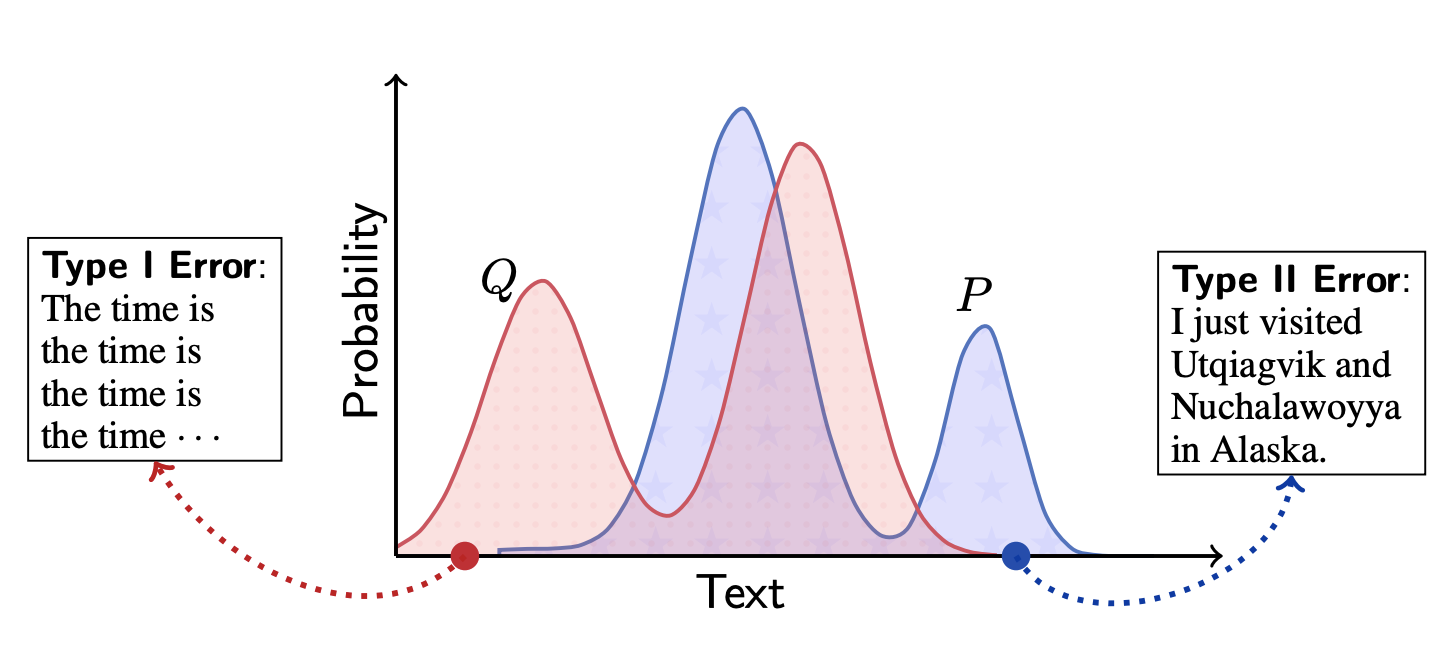In the figure, we plot the probability of occurrence of the model and the Human text, which is depicted as a probability distribution. The KL-divergence is a metric that measures the gap between probability distributions, and the larger it is, the more different the two distributions are. The KL-divergence is non-negative, and its value differs depending on which probability distribution is taken as the reference.

At MAUVE, we have made two major efforts in implementing KL-divergence. We will explain them in this section.

### Devise 1. make probability distribution by using text vector

When we use KL-divergence in NLP, we often create a probability distribution for Vocabulary. However, in this study, we generate text vectors by a pre-training model (GPT-2), perform clustering, and create a probability distribution based on the vectors. By doing so, we succeeded in incorporating more contextual meanings than measuring KL-divergence by Vocabulary, and at the same time, we reduced the amount of computation. The concrete procedure is as follows.

1. Sampling the text of generated text and Human text respectively (5000 in the paper)
2. Make each text a vector using GPT-2
3. Mix text vectors and cluster them with k-means (500 clusters in the paper)
4. Create probability distributions of clusters for generated text and human text respectively.

If you are interested, please refer to the Appendix.

### Devise 2. measure KL-divergence with mixture distribution

One of the important properties of KL-divergence is that the values may diverge if the distributions are too different. Therefore, KL-divergence is not suitable as an evaluation metric. In this study, we do not measure KL-divergence directly between the two distributions, i.e., generated and human text, but between the mixture of the two distributions and each of the two distributions. The KL-divergence of Human text is calculated by the following formula.

$\mathrm{KL}\left(P \mid R_{\lambda}\right)=\sum_{\boldsymbol{x}} P(\boldsymbol{x}) \log \frac{P(\boldsymbol{x})}{R_{\lambda}(\boldsymbol{x})}$

where $R_\lambda$ is the mixture distribution of generated text and Human text (or $Q(\boldsymbol{x})$ for normal KL-divergence). The $R_\lambda$ is generated as follows.

$R_{\lambda}=\lambda P+(1-\lambda) Q$

The $\lambda$ is a hyperparameter that takes a value range of [0,1]. MAUVEuses the divergence curve obtained by moving $\lambda$ in the range [0,1 ]. the formula of the divergence curve is as follows.

$\mathcal{C}(P, Q)=\left{\left(\exp \left(-c \mathrm{KL}\left(Q \mid R_{\lambda}\right)\right), \exp \left(-c \mathrm{KL}\left(P \mid R_{\lambda}\right)\right)\right): R_{\lambda}=\lambda P+(1-\lambda) Q, \lambda \in(0,1)\right}$

Here, c is a hyperparameter for scaling with c>0, and exp is processed to make the value range of KL-divergence [0,1]. The figure of KL-divergence to mixture distribution from the view of generated text and Human text is as follows.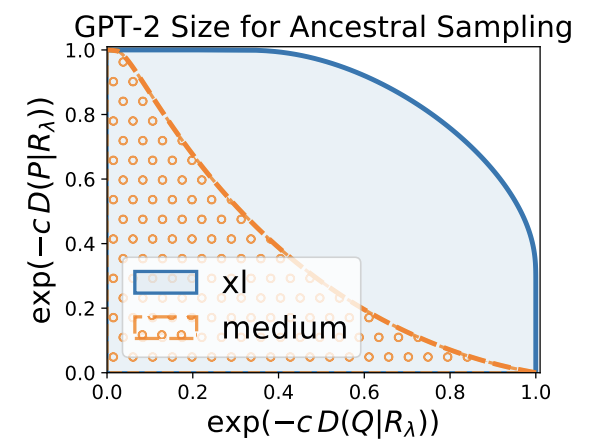The figure is a bit complicated to read, but the gap to the mixture distribution from the generated text shows the value of $\lambda$ on the horizontal axis and the value of $\exp\left(-c KL\left(Q\mid R_{\lambda}\right)}\right)$ on the vertical axis. On the other hand, if we focus on the gap to the mixture distribution from the Human text, the vertical axis shows the value of $\lambda$ and the horizontal axis shows the value of $\exp \left(-c KL\left(P \mid R_{\lambda}\right)\right)$.

The area under this divergence curve is the MAUVE score. In the above figure, we are comparing the scores of text generated by GPT-2 xl and medium, and the score of xl is larger than that of the medium, which empirically justifies MAUVE.

## experiment

In the experimental section, in addition to some empirical evaluations, we perform a manual evaluation.

the task is a text completion task in the domain of web text, news, and stories. Given the first word sequence, the task is to predict the rest of the word sequence. For the sake of the task, we assume that the original word sequence is the human text, but the task does not have a clear correct answer.
• Decoding algorithm ( reference ): We consider three decoding algorithms. In general, the accuracy of the model is in the order of Greedy decoding<Ancestral sampling<Nucleus sampling.
• Greedy decoding
At each decoding step, always choose the word with the highest probability of occurrence.
• Ancestral sampling
Selects the next word probabilistically according to the probability distribution of the language model.
• Nucleus sampling
Truncate all but the top p words, and select the next word according to the distribution scaled by the remaining words.

### Does the accuracy decrease as the sentence length increases?

The longer the text generated by the model, of course, the worse the accuracy (i.e., deviation from Human text). We experiment to see if automatic evaluation metrics, including those from previous studies, can capture this phenomenon.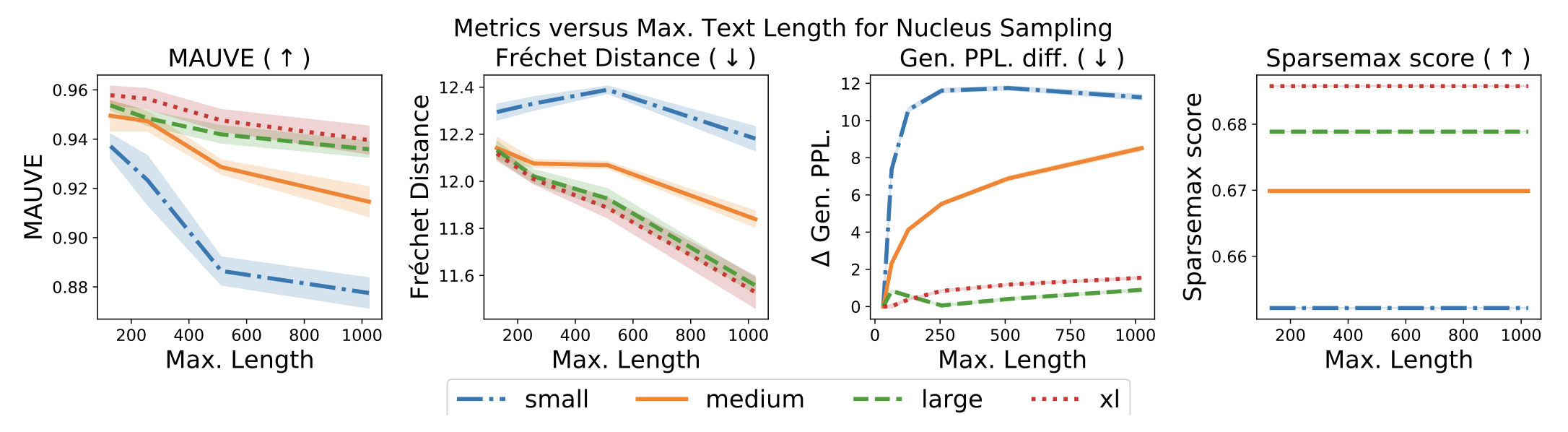You can see that MAUVE on the left is the only one that captures the change. No other indicator has been able to capture changes in all model sizes.

### Can we capture differences in decoding algorithms and model sizes?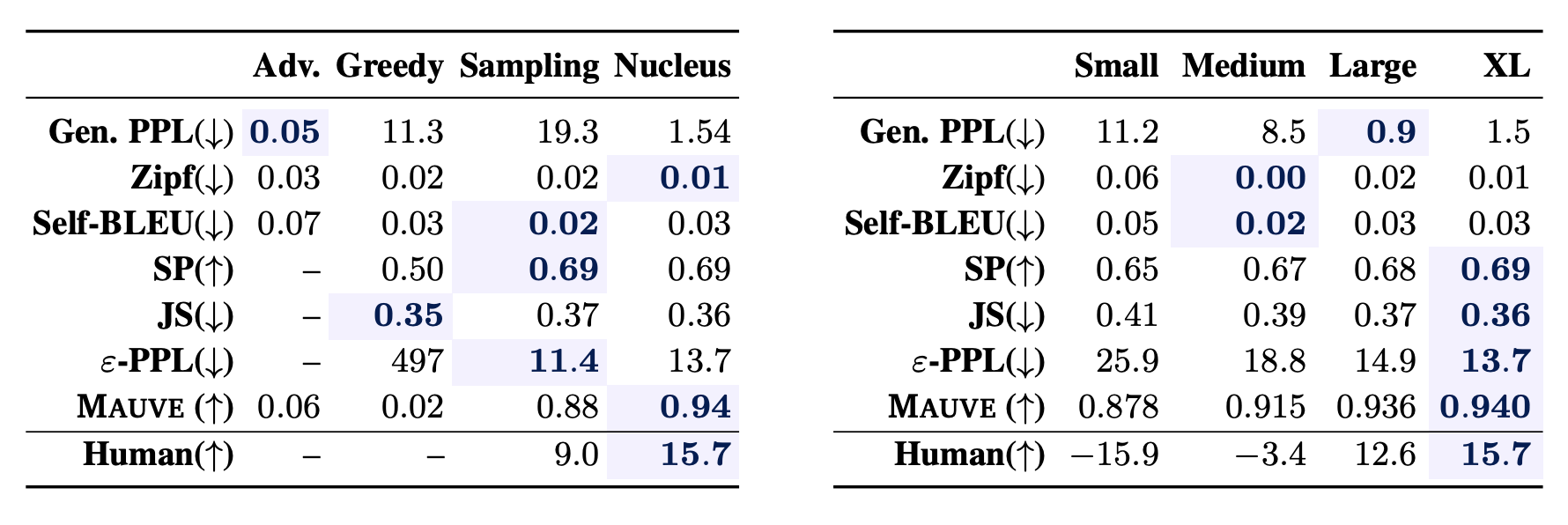The left table shows that MAUVE is scored in the order of Greedy decoding<Ancestral sampling<Nucleus sampling. The right table also shows that MAUVE scores are higher for the GPT-2 model sizes Small, Medium, Large, and XL.

### Is it correlated with manpower assessment?

So far, the evaluation has been empirical, but we will see if it correlates with the most important human evaluation. We employ three metrics that are often used in open-ended task evaluation: Human-like, Interesting, and Sensible, and annotate them against the text generated by the model. The correlation coefficients between each metric and the human scores are shown in the table below.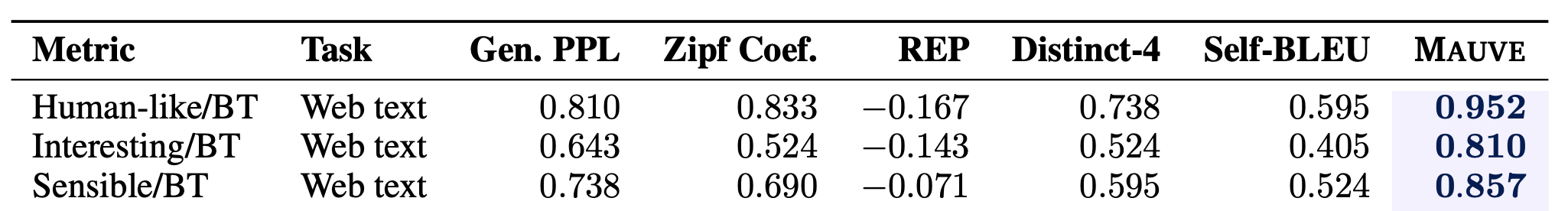MAUVE shows a significantly higher correlation than previous studies. It can be said that the high correlation allows us to evaluate the open-ended task with high accuracy.

## summary

In this article, we introduced MAUVE, which can evaluate the quality of the generated text with high accuracy.

In addition to the accuracy, it seems to be quite easy to use as it is already available through pip and does not require training of the model. It may well become a new de facto standard as an evaluation metric for open-ended tasks.

On the other hand, the large number of high paras, such as the number of sampling and the selection of clustering algorithm, may be a barrier when we want to construct the index with new data, but this paper quite carefully experiments in the Appendix, and that may have contributed to the high evaluation. Automatic evaluation of open-ended tasks has not been conclusive yet, and the way the scores have increased shows that there has been a lot of improvement in recent years, so this is one of the research areas that we are looking forward to seeing how it develops in the future.

If you have any suggestions for improvement of the content of the article,